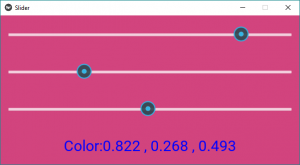Related Articles
Python | Multiple Sliders widgets Controlling Background Screen or WindowColor in Kivy
• Last Updated : 07 Feb, 2020

Prerequisite – Slider in Kivy

Kivy is a platform independent GUI tool in Python. As it can be run on Android, IOS, linux and Windows etc. It is basically used to develop the Android application, but it does not mean that it can not be used on Desktops applications.

In this article, we will learn How we can control the background color in kivy that means if we slide the slider the color of window changes accordingly.

There are many different methods by which you can make this. Let’s see one simple approach.

```Basic Approach to make it is very simple:
1) import kivy
2) import kivyApp
3) import BoxLayout
4) set minimum version(optional)
5) Extend the class
6) set up .kv file :
-> Set orientation
-> Set slider color
-> Create canvas.before property
-> Create Sliders
-> Create label
7) Return layout
8) Run an instance of the class```

How multiple Sliders Controlling Background Color in Kivy ?

 `# main.py to manipulate the window ``# color or screen clour in kivy`` ` `# base Class of your App inherits from the App class.  ``# app:always refers to the instance of your application  ``from` `kivy.app ``import` `App`` ` `# BoxLayout arranges children in a vertical or horizontal box. ``# or help to put the childrens at the desired location. ``from` `kivy.uix.boxlayout ``import` `BoxLayout `` ` `# creating the root widget used in .kv file ``class` `MultipleSliderWidget(BoxLayout):``    ``pass`` ` `# class in which name .kv file must be named Slider.kv.``# or creating the App class``class` `Multiple_Slider(App):``    ``def` `build(``self``):``        ``# returning the instance of SliderWidget class ``        ``return` `MultipleSliderWidget()`` ` `# run the app    ``if` `__name__ ``=``=` `'__main__'``:``    ``Multiple_Slider().run()`

Now the .kv file of the above code is –

 `# Multiple_Slider.kv file of the main.py file.`` ` `#.kv file to manipulate the window colour.``:``     ` `    ``# giving the orientation of Slider``    ``orientation: ``"vertical"`` ` `    ``# initially providing this colour to window``    ``slider_colors: ``0.5``, ``0.5``, ``0.5`` ` `    ``# executed before the canvas group.``    ``canvas.before:``        ``Color:``            ``rgb: root.slider_colors``        ``Rectangle:``            ``pos: root.pos``            ``size: root.size``             ` `    ``# creating the Slider``    ``Slider:``        ``min``: ``0`  `# minimum value of Slider``        ``max``: ``1` `# maximum value of Slider``        ``value: ``0.5`  `# initial value of Slider``         ` `        ``# when slider moves then to increase value``        ``on_value: root.slider_colors[``0``] ``=` `self``.value;`` ` `    ``Slider:``        ``min``: ``0``        ``max``: ``1``        ``value: ``0.5``        ``on_value: root.slider_colors[``1``] ``=` `self``.value`` ` `    ``Slider:``        ``min``: ``0``        ``max``: ``1``        ``value: ``0.5``        ``on_value: root.slider_colors[``2``] ``=` `self``.value`` ` `    ``# Adding The label ``    ``Label:``        ``font_size: ``"30sp"``        ``# the for loop is for continuously changing``        ``# the clour as slider value changes``        ``text: ``"Color:"` `+` `", "``.join([``"%.3f"` `%``(i) ``for` `i ``in` `root.slider_colors]) ``        ``color: ``0``, ``0``, ``1``, ``1`

Output:Videos Output:

Attention geek! Strengthen your foundations with the Python Programming Foundation Course and learn the basics.

To begin with, your interview preparations Enhance your Data Structures concepts with the Python DS Course. And to begin with your Machine Learning Journey, join the Machine Learning – Basic Level Course

My Personal Notes arrow_drop_up